# Examination Questions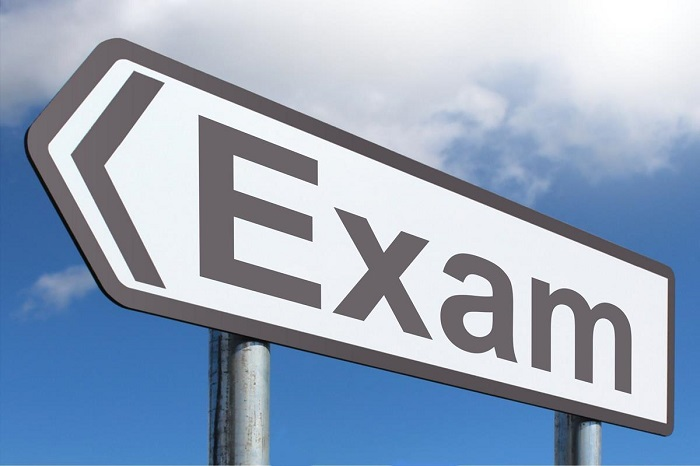This section of the website has practice examination questions for SL Mathematics: Applications and Interpretation for you to try. They all come with video solutions and are split up by the same subtopics we have on the site. There is no substitute for lots of practice. You can can lots of quickfire practice with out  Question Bank on your lap top on on your phone via the app (inthinking revision app) and here you find the more involved exam style questions! Good luck!

Dont forget that we also have

1. Interactive self checking quizzes onn every page - you can see them all here on this Practice Questions page
2. A huge  Question Bank  with over 1700 practice questions you can do on a lap top or the app!

#### Number & Algebra Exam Questions

The following are exam style questions for the sub-topics in the number and algebra unit. There are questions with video solutions here for here for Standard Index Form, Arithmetic Sequences and Geometric Sequences, Exponents and Logarithms.

#### Functions - Exam Style Questions

The following are exam style questions for the sub-topics in the functions unit. There are questions with video solutions here for here for Introduction, Key Features and Graphing, Linear Models, Quadratic Models, Exponential Models and Trigonometric...

#### Geometry & Trigonometry Exam Style Questions

The following are exam style questions for the sub-topics in the functions unit. There are questions with video solutions here for here for Volume and Surface area, right angled trigonometry, Nin right angled trigonometry, applications of trigonometry,...

#### Statistics & Probability Exam Questions

The following are exam style questions for the sub-topics in the functions unit. There are questions with video solutions here for here for Statistical concepts, cumulative frequency and box plots, central tendency and dispersion, bivariate...

#### Calculus Exam questions

The following are exam style questions for the sub-topics in the functions unit. There are questions with video solutions here for here for introduction, tangents and normals, stationary points and optimisation and integration an the trapezoidal...

•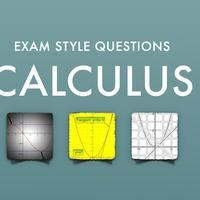#### Calculus Exam questions

The following are exam style questions for the sub-topics in the functions unit. There are questions with video solutions here for here for Statistical concepts, cumulative frequency and box plots, central tendency and dispersion, bivariate...

•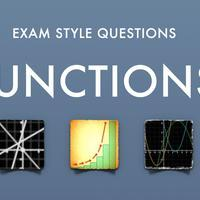#### Functions - Exam Style Questions

The following are exam style questions for the sub-topics in the functions unit. There are questions with video solutions here for here for Introduction, Key Features and Graphing, Linear Models, Quadratic Models, Exponential Models and Trigonometric...

•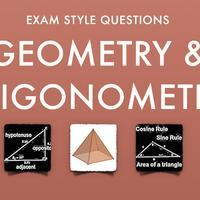#### Geometry & Trigonometry Exam Style Questions

The following are exam style questions for the sub-topics in the functions unit. There are questions with video solutions here for here for Volume and Surface area, right angled trigonometry, Nin right angled trigonometry, applications of trigonometry,...

•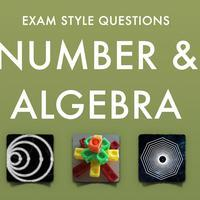#### Number & Algebra Exam Questions

The following are exam style questions for the sub-topics in the number and algebra unit. There are questions with video solutions here for here for Standard Index Form, Arithmetic Sequences and Geometric Sequences, Exponents and Logarithms.

•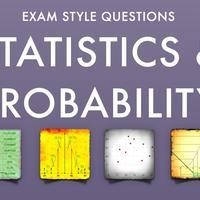#### Statistics & Probability Exam Questions

The following are exam style questions for the sub-topics in the functions unit. There are questions with video solutions here for here for Statistical concepts, cumulative frequency and box plots, central tendency and dispersion, bivariate...